# Computing Volumes of Surfaces of Revolution

Everybody knows that the voluem of a solid box is

volume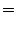length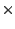widthheightMore generally, the volume of cylinder is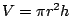(cross sectional area times height). Even more generally, if the base of a prism has area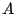, the volume of the prism is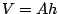.

But what if our solid object looks like a complicated blob? How would we compute the volume? We'll do something that by now should seem familiar, which is to chop the object into small pieces and take the limit of approximations.

[[Picture of solid sliced vertically into a bunch of vertical thin solid discs.]]

Assume that we have a function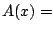cross sectional area at $x$The volume of our potentially complicated blob is approximately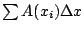. Thus

 volume of blob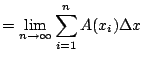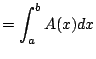Example 3.2.1   Find the volume of the pyramid with height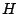and square base with sides of length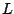.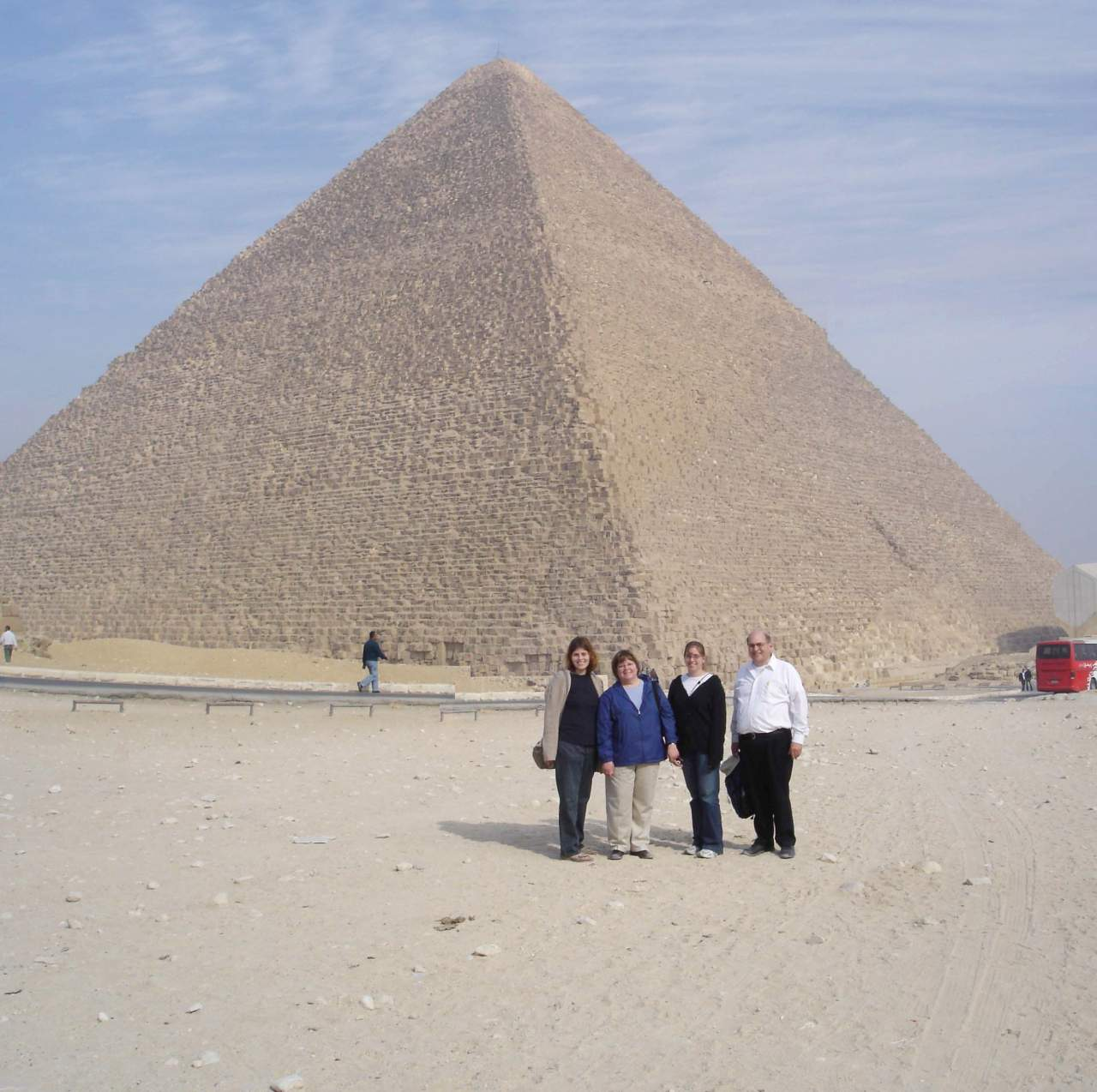For convenience look at pyramid on its side, with the tip of the pyramid at the origin. We need to figure out the cross sectional area as a function of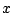, for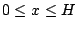. The function that gives the distance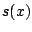from theaxis to the edge is a line, with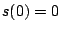and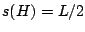. The equation of this line is thus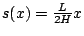. Thus the cross sectional area is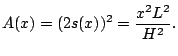The volume is then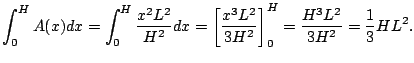Today: Quiz! Next: Polar coordinates, etc. Questions:? Recall: Find volume by integrating cross section of area. (draw picture)

Example 3.2.2   Find the volume of the solid obtained by rotating the following region about theaxis: the region enclosed by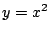and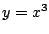between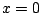and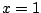.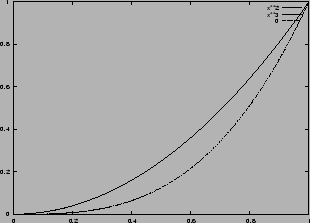The cross section is a washer'', and the area as a function ofis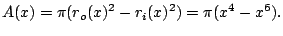The volume is thus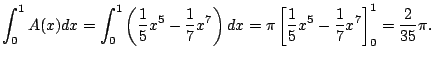Example 3.2.3   One of the most important examples of a volume is the volume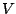of a sphere of radius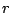. Let's find it! We'll just compute the volume of a half and multiply by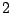.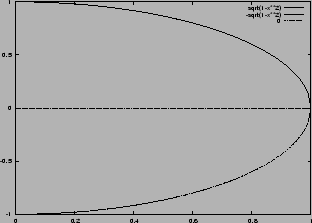The cross sectional area is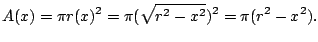Then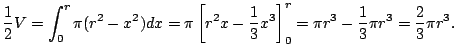Thus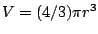.

Example 3.2.4   Find volume of intersection of two spheres of radius, where the center of each sphere lies on the edge of the other sphere.

From the picture we see that the answer is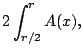where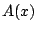is exactly as in Example 3.2.3. We have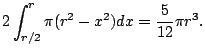William Stein 2006-03-15